# Perron numbers that satisfy Fermat’s equation

Pietro Paparella
Notes on Number Theory and Discrete Mathematics
Print ISSN 1310–5132, Online ISSN 2367–8275
Volume 27, 2021, Number 3, Pages 119—122
DOI: 10.7546/nntdm.2021.27.3.119-122

## Details

### Authors and affiliations

Pietro PaparellaDivision of Engineering and Mathematics, University of Washington Bothell
18115 Campus Way NE, Bothell, WA 98011, United States

### Abstract

In this note, it is shown that if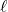andare positive integers such that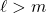, then there is a Perron number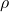such that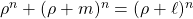. It is also shown that there is an aperiodic integer matrix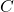such that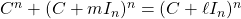.

### Keywords

• Perron number
• Fermat equation
• Integer matrix
• Aperiodic matrix

• 11D41
• 15B36

### References

1. Arnold, M., & Eydelzon, A. (2019). On matrix Pythagorean triples. The American Mathematical Monthly, 126(2), 158–160.
2. Brenner, J. L., & de Pillis, J. (1972). Fermat’s equation Ap + Bp = Cp for matrices of integers. Mathematics Magazine, 45, 12–15.
3. Brualdi, R. A., & Ryser, H. J. (1991). Combinatorial Matrix Theory. Cambridge: Cambridge University Press.
4. Horn, R. A., & Johnson, C. R. (1990). Matrix Analysis. Cambridge: Cambridge University Press.
5. Lind, D. A. (1983). Entropies and factorizations of topological Markov shifts. Bulletin of the American Mathematical Society, 9(2), 219–222.
6. Lind, D. A. (1984). The entropies of topological Markov shifts and a related class of algebraic integers. Ergodic Theory and Dynamical Systems, 4, 283–300.

## Cite this paper

Paparella, P. (2021). Perron numbers that satisfy Fermat’s equation. Notes on Number Theory and Discrete Mathematics, 27(3), 119-122, doi: 10.7546/nntdm.2021.27.3.119-122.